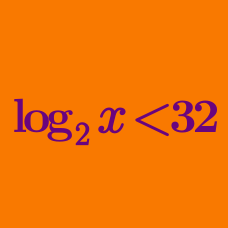Algebra

# Logarithmic Inequalities - Base < 1

What is the range of $$x$$ that satisfies the logarithmic inequality $\log_{\frac{1}{3}}(5x-7) \geq \log_{\frac{1}{3}}(3x+5) ?$

What is the maximum integer value of $$x$$ that satisfies the logarithmic inequality $\log_{\frac{1}{2}}(x-4) > \log_{\frac{1}{4}}(2x-5) ?$

What is the range of $$x$$ that satisfies the logarithmic inequality $\log_{0.4}(x^2-23) - \log_{0.4}(x-5) < \log_{0.4}{7} ?$

What is the range of $$x$$ that satisfies the logarithmic inequality $\log_{0.5}{(2x+61)} > -3 ?$

What is the range of $$x$$ that satisfies the logarithmic inequality $\log_{\frac{1}{5}}x > \log_{\frac{1}{7}}x ?$

×

Problem Loading...

Note Loading...

Set Loading...